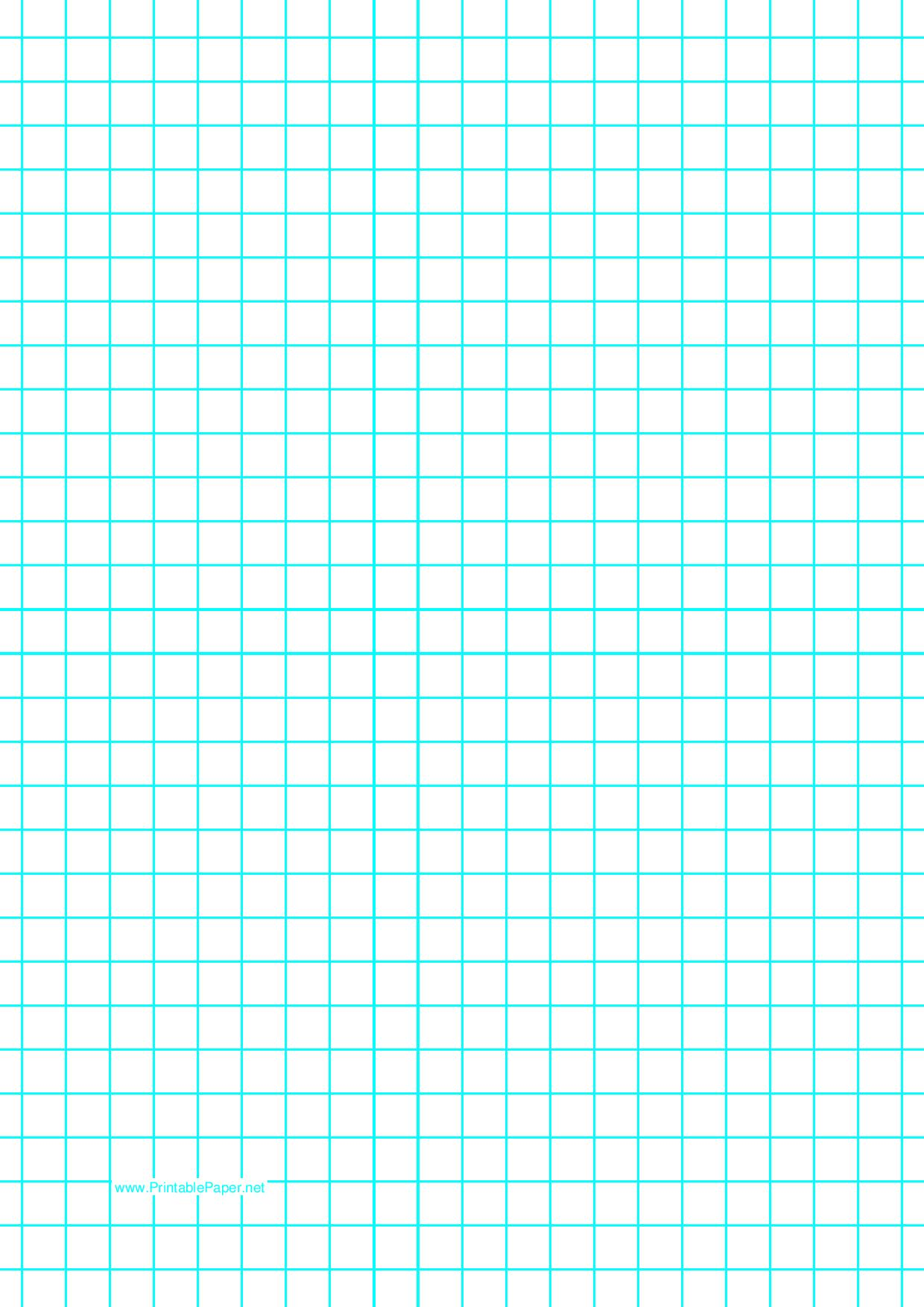# Printable Centimeter Graph Paper or CM Grid Paper PDF

A centimeter graph paper comes in handy when it comes to learning mathematical concepts such as calculus or geometry. Students or kids in the schools often use this graph paper to learn or understand the various math concepts. Apart from that, this graph paper can be used for plotting a line, charting, or even design. 1 cm graph paper is essential for all grades, below you can check out the collection of centimeter graph paper or CM graph paper templates available for free download in a pdf format.

If you are a student of mathematics or engineering then doc heck out the CM graph paper templates here and download a suitable one for your use. A grid paper that is ready to use by any student for solving math equations, recording data, etc. Different types of printable centimeter graph papers are shared here for free download in a printable format.

## Square Centimeter Graph Paper

If you are wondering why this graph paper is called Centimeter Graph Paper then please note that the grids in this graph paper are 1 cm only and the magnitude of the paper is given 8.5″ x 11″. This graph paper is one of the most commonly used graph papers. The grids are in square format as you can see in the template below.

This type of graph paper is used in geometry, science, and other working places. A graph paper is one of the most essential documents that is used by students for their work purposes.## Printable Centimeter Grid Paper

Printable graph papers are available here for free download in a pdf format. You can take a print-out of the graph paper easily for free. Making graph paper from scratch consumes a lot of time and effort, this is why it makes more sense to download a template and make a graph paper for your use. We have tried to share only the high quality of graph papers that will give you a good quality of print-out easily.Another type of graph paper:

### Centimeter Graph Paper Template

Multiple templates are available here. Please note that each template has something to offer. Therefore, you need to choose your graph paper wisely depending on the type of work you are involved in. If you need the length of the grids in centimeters then you can use it like that and if you need the length of the grids in mm then you can focus on the lines with the length in mm.